Courses

# NCERT Exemplar - Work and Energy Class 9 Notes | EduRev

## Class 9 : NCERT Exemplar - Work and Energy Class 9 Notes | EduRev

The document NCERT Exemplar - Work and Energy Class 9 Notes | EduRev is a part of the Class 9 Course Science Class 9.
All you need of Class 9 at this link: Class 9

Q.1. Avinash can run with a speed of 8 ms-1 against the frictional force of 10 N, and Kapil can move with a speed of 3 ms-1 against the frictional force of 25 N. Who is more powerful and why?
Ans: Power of Avinash, P1 = F1. v1 = 10 x 8 = 80 watt
Power of Kapil, P2 = F2.v2 = 25 x 3 = 75 watt
So Avinash is more powerful the Kapil.

Q.2. Can any object have mechanical energy even if its momentum is zero? Explain.
Ans: Yes, when a body is thrown up, P.E. (in the form of mechanical energy) is non-zero at the highest point of a projectile, even if K.E. is zero due to zero velocity.

Q.3. Can any object have momentum even if its mechanical energy is zero? Explain.
Ans: No, as zero energy means both P.E. and K.E are zero. This makes velocity as well as momentum zero.

Q.4. The power of a motor pump is 2 kW. flow much water per minute the pump can raise to a height of 10 m? (g = 10 ms-2)
Ans:
As m is raised to a height 'h'
P.E. gained = mgh.
Power,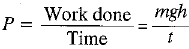Thus,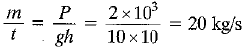∴ Mass of water pumped per minute
= 20 x 60 = 1200 kg

Q.5. The weight of a person on a planet A is about half that on the earth. He can jump upto 0.4 m height on the surface of the earth. How high he can jump on the planet A?
Ans:
By the law of conservation of energy,
mg1h1 = mg2h2
[where, g1 = force of gravity on earth,
g2 = force of gravity on planet A.]
As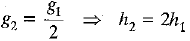Therefore, the height of jump of the person on planet A will be, h2 = 2h1 = 2 x 0.4 = 0.8 m.

Q.6. The velocity of a body moving in a straight line is increased by applying a constant force F, for some distance in the direction of the motion. Prove that the increase in the kinetic energy of the body is equal to the work done by the force on the body.
Ans:
Work done = F.s = ma.s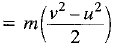[∵ v2 = u2 + 2as]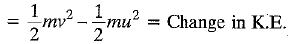Q.7. Is it possible that an object is in the state of accelerated motion due to external force acting on it, but no work is being done by the force? Explain it with an example.
Ans: Yes, it is possible. As the body moves in a circle, it experiences centripetal acceleration towards the centre and the displacement is along the tangent at any point of the circle. Since, force and displacement are perpendicular, work done is zero.

Q.8. A ball is dropped from a height of 10 m. If the energy of the ball reduces by 40% after striking the ground, how much high can the ball bounce back? (g = 10 ms-2)
Ans: 40% of the initial energy is lost. So, the ball will bounce back with 1/6th of the initial height with the remaining 60% energy, i.e. 6 m.

Q.9. A light object and a heavy object have the same momentum. Find out the ratio of their kinetic energies. Which one has a larger kinetic energy?
Ans: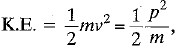where p = mv.
When momentum is same,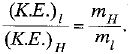Lighter mass will have larger kinetic energy

Q.10. A girl having mass of 35 kg sits on a trolley of mass 5 kg. The trolley is given an initial velocity of 4 ms-1 by applying a force. The trolley comes to rest after travelling a distance of 16 m.
(a) How much work is done on the trolley?

(b) How much work is done by the girl?
Ans: Given: = 4 ms-1, v = 0, s = 16 m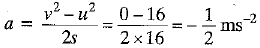(Neglecting -ve sign)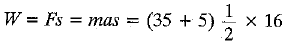= 20 x 16 = 320 joule
So, (a) 320 joule of work is done on the trolley
(b) work done by the girl is zero.

Q.11. Four men lift a 250 kg box to a height of 1 m and hold it without raising or lowering it.
(a) How much work is done by the men in lifting the box?
(b) How much work do they do in just holding it?
(c) Why do they get tired while holding it?
(g = 10 ms-2)
Ans: (a) Work done in lifting = mgh
= 250 x 10 x 1
= 2500 joule
(b) Work done is zero as displacement is zero,
(c) Muscular energy is used up in holding. So, they get tired on just holding.

Q.12. What is power? How do you differentiate kilowatt from kilowatt hour? The Jog Falls in Karnataka state are nearly 20 m high. 2000 tonnes of water falls from it in a minute.
Calculate the equivalent power if all this energy can be utilized. (g = 10 ms-2)
Ans:
Power is the rate of doing work. Kilowatt is a unit for power while kilowatt hour is a unit for electrical energy consumed.
m = 2000 x 103 kg, h = 20 m
t = 1 min = 60 s.
Power of falling water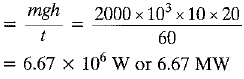Q.13. How is the power related to the speed at which a body can be lifted? How many kilograms will a man working at the power of 100 W, be able to lift at constant speed of 1 m s-1 vertically? (g = 10 ms-2)
Ans:
Power = Force x Velocity or P = Fv
P = 100 W and v = 1 m s-1, F = P/v = 100 N
F = mg or m = F/g = 100 N/10 ms2 = 10 kg
Therefore, 10 kg can be lifted by a man.

Q.14. Compare the power at which each of the following is moving upwards against the force of gravity.
(a) a butterfly of mass 1.0 g that flies upward at a rate of 0.5 ms-1.
(b) a 250 g squirrel climbing up on a tree at a rate of 0.5 ms-1. (g = 10 ms-2)
Ans:
(a) Since, P = Fv = mgv
Given: m = 1 g = 10-3 kg, v = 0.5 ms-1
Power of butterfly = 10-3 x 10 x 0.5
= 5 x 10-3
= 0.005 watt
(b) Given: m = 250 g = 250 x 10-3 kg,
v = 0.5 ms-1
Power of squirrel = 250 x 10-3 x 10 x 0.5
= 1250 x 10-3 = 1.25 watt
Therefore, power of the squirrel is more than that of butterfly.

Offer running on EduRev: Apply code STAYHOME200 to get INR 200 off on our premium plan EduRev Infinity!

## Science Class 9

95 videos|223 docs|135 tests

,

,

,

,

,

,

,

,

,

,

,

,

,

,

,

,

,

,

,

,

,

;